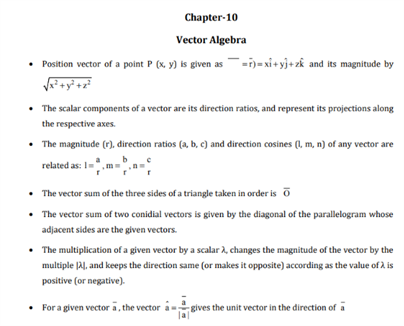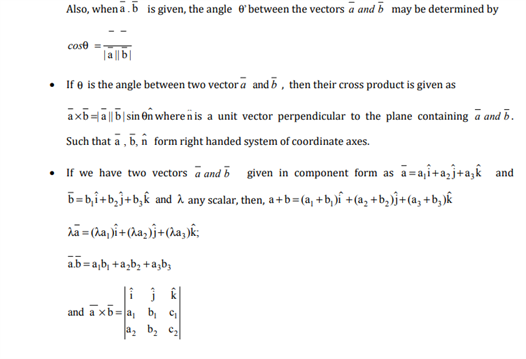# Maths Revision Notes for Class 12 Chapter 10 Vector Algebra.

The 10th chapter for class 12 Maths is titled as Vector Algebra. The concept of vector algebra in mathematics is defined as the basic operations used in vector addition and scalar multiplication. The different vectors that are listed in this chapter are:

• Zero Vector
• Unit Vector
• Equal Vector
• Collinear Vector
• Coplanar Vector

Other major concepts that are taught is the position vector of a point and Algebra of Vectors. Students here can learn about the addition and multiplication of vectors, among other topics.

Thus, these are the CBSE revision notes for chapter 10 Vector Algebra, available below:You may also want to read:

 NCERT Related Articles NCERT Solutions for Class 12 NCERT Solutions for Class 11 NCERT Solutions for Class 10 NCERT Solutions for Class 12 Maths NCERT Solutions for Class 11 Maths NCERT Solutions for Class 10 Maths NCERT Solutions for Class 12 Physics NCERT Solutions for Class 11 Physics NCERT Solutions for Class 10 Science NCERT Solutions for Class 12 Chemistry NCERT Solutions for Class 11 Chemistry NCERT Solutions for Class 6 NCERT Solutions for Class 12 Biology NCERT Solutions for Class 11 Biology NCERT Solutions for Class 6 Maths NCERT Solutions for Class 9 NCERT Solutions for Class 8 NCERT Solutions for Class 6 Science NCERT Solutions for Class 9 Maths NCERT Solutions for Class 8 Maths NCERT Solutions for Class 4 NCERT Solutions for Class 9 Science NCERT Solutions for Class 8 Science NCERT Solutions for Class 4 Maths NCERT Solutions for Class 5 NCERT Solutions for Class 7 NCERT Solutions for Class 7 Maths NCERT Solutions for Class 4 Science NCERT Solutions for Class 5 Maths NCERT Solutions for Class 7 Science NCERT Solutions NCERT Books NCERT Solutions for Class 5 Science